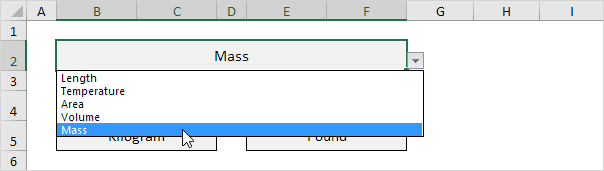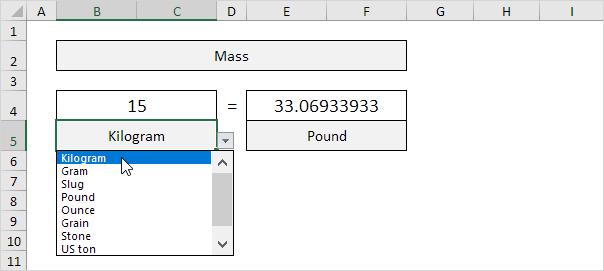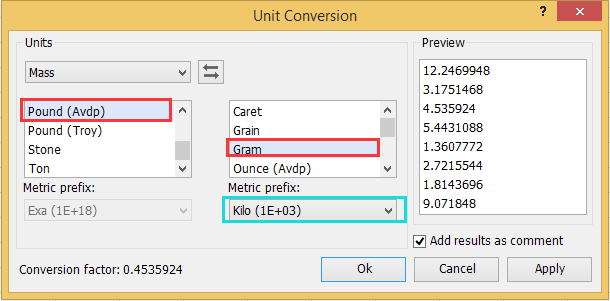# How to convert pound to Kgs in Excel

The pound is the name of several units of mass, ranging from 300 to 600 grams. Kilogram KG is the SI unit for mass. 1 lbs (pound) = 0.45359 kgs or 1Kg= 2.20462. Having known how kilograms and pounds relate, it is easy to convert from one unit to the other.

## Conversion using excel

Conversion of Pound to kilogram can also be done using excel.it is done by following the simple steps;

Let us take an example of 10kgs and see how the conversion

1. The first thing during conversion from kg to lbs is to multiply by 2.20462. =A1*2.20462.2. To convert lbs to kgs you will divide by 2.20462. Use the formula A1/2.20462Alternative,

You can use also the convert function in excel from kgs to lbs. the function used in the conversion is =CONVERT(A1, "kg", " lbm")There are three arguments in the convert function (number, from_unit, and to_unit). lbm is the unit of abbreviation that stands for pound mass.

3. This convert function in excel can also be used to convert from lbs to kg.To quickly converter from kg to lbs and vice versa, download a free unit converter which will simplify things

4. From the category drop list select the type of unit you are interested in.5. Have a number as an input and select the correct mass unit.## How the unit converter works

When you update a cell, the unit converter leverages worksheet change events to automatically run VBA code (B2, B4, B5, E4, or E5). The VBA code converts one measuring unit to another and then utilizes the VLOOKUP function to get the right unit abbreviations (kg, g, lbm, ozm, etc.) saved on the second worksheet.

## How to convert Kgs to lbs using Kutools for Excel

When you use excel in the conversions it is paramount to recall the formula and the relation of the units. When using Kutools for Excel allows you to carry conversions between multiple mass units.

## steps:

Once you have Kutool on your computer the following steps are followed in conversion;

1. Choose the range of data you want to convert and click on Kutool

2. On Kutool click on content then unit conversion.3. On the unit conversion dialog, from the drop-down menu, select mass under the unit section.

4. Select the unit you want to convert.5. To exchange the units of conversion quickly, click the swap unit button and click okay to accept the changes.

## Converting Pounds to Kgs

1. Select Pound (Avep) from the list on the left side and then the gram unit on the right side. On the metric prefix select Kilo (1E+03) just below the gram.2. You can use cell comments by checking the additional results as a comment option in the unit conversion dialogue to show the converting results.

Click okay after selecting the scope of conversion.

Conversion between pounds to kg is easy all it needs is understanding the relationship between the two units. The use of Kutool has made it easy since it automatically converts and does not need to remember the relation.